# Artificial neural network

11 Mar 2017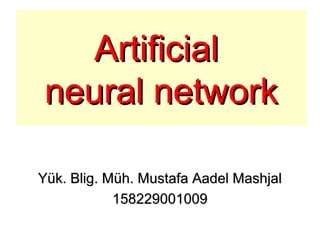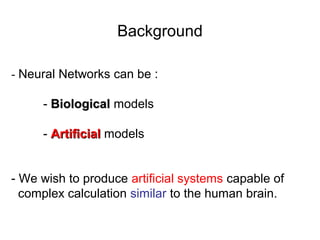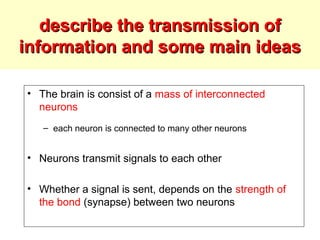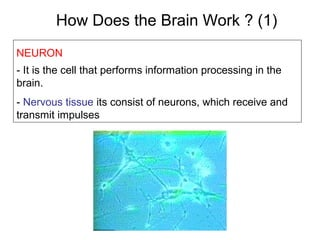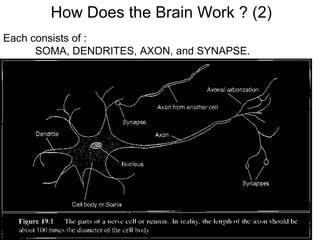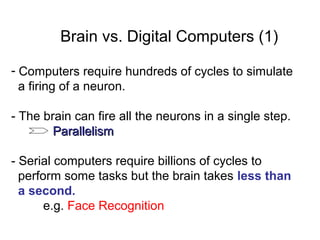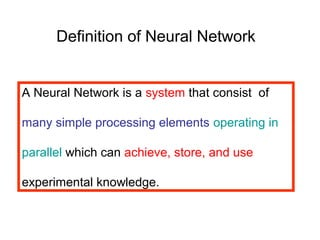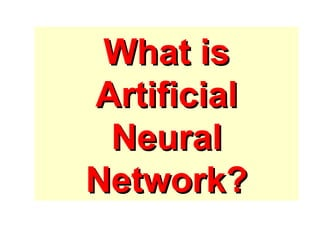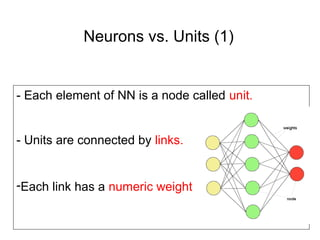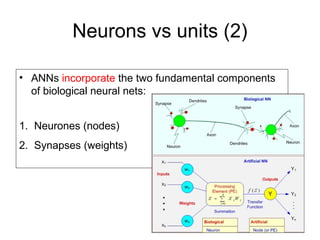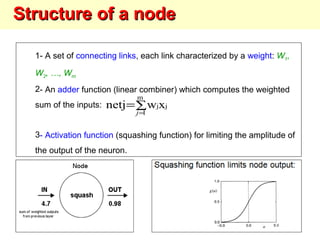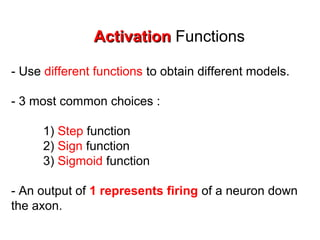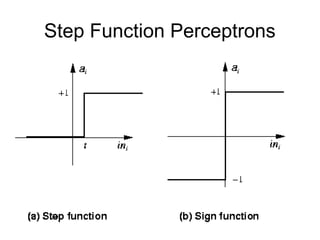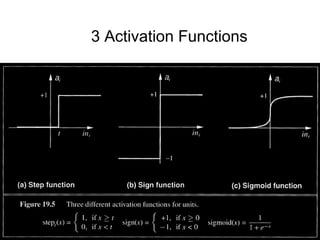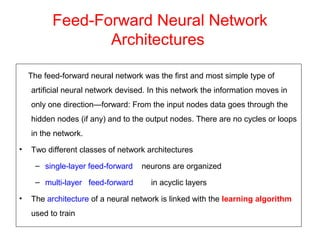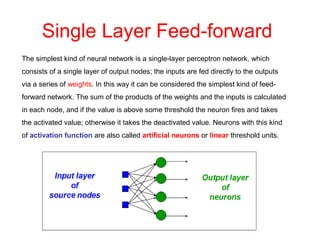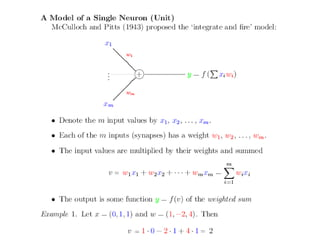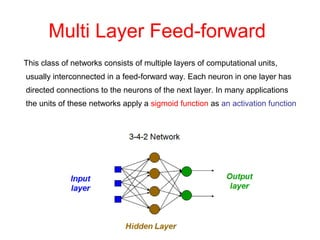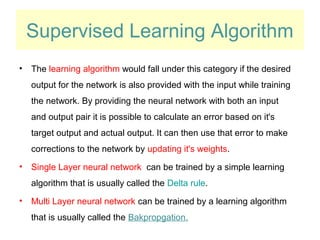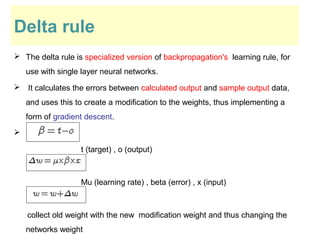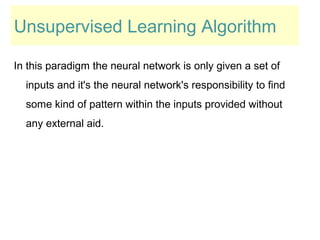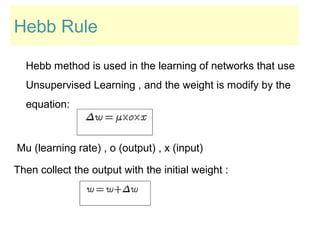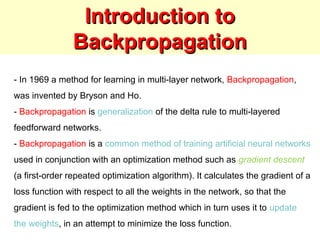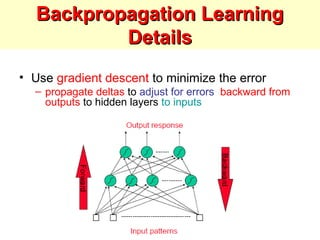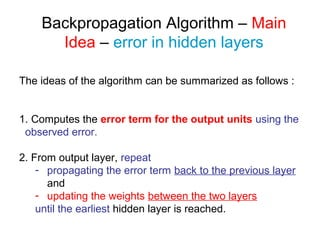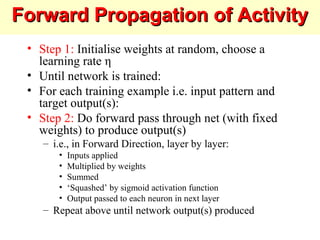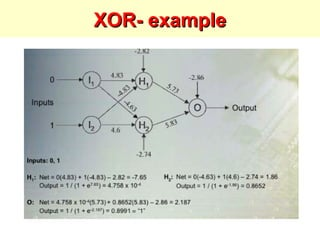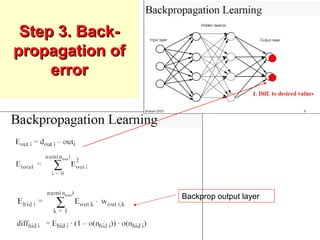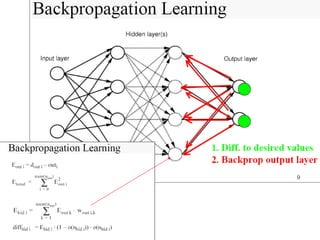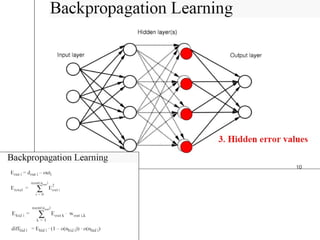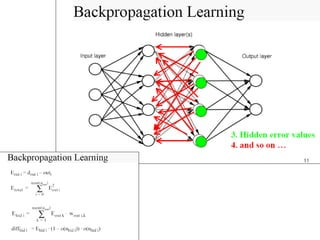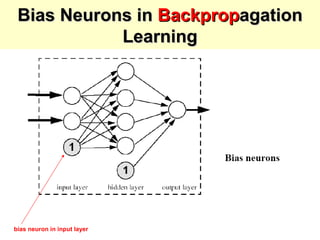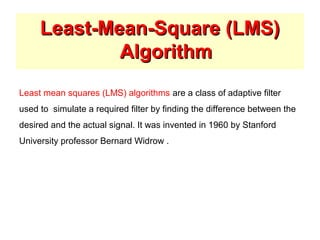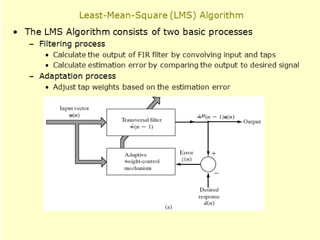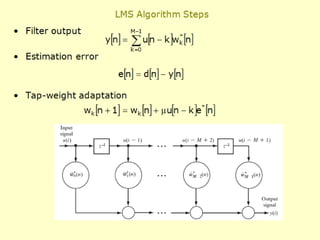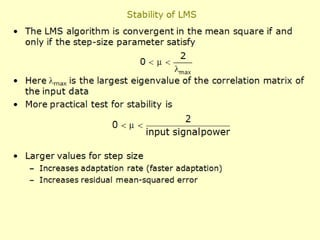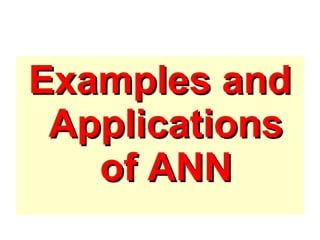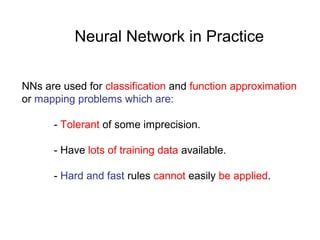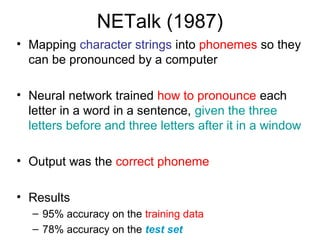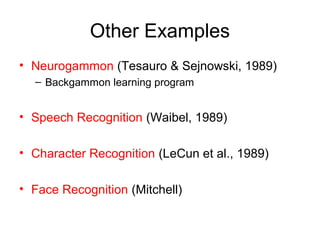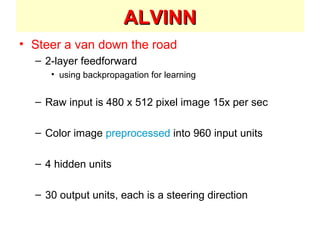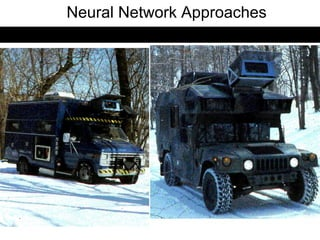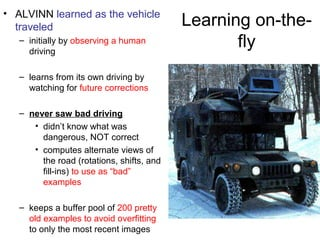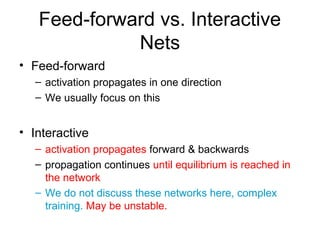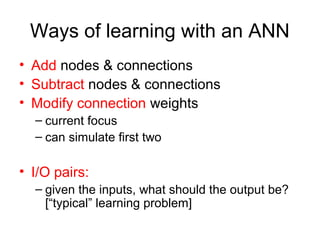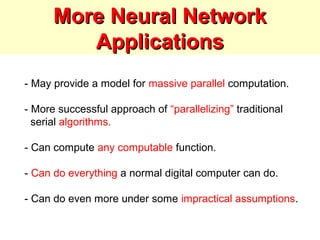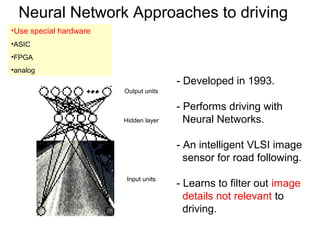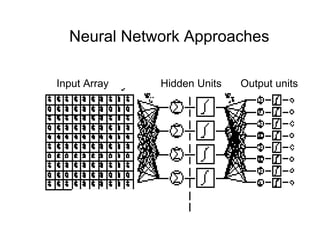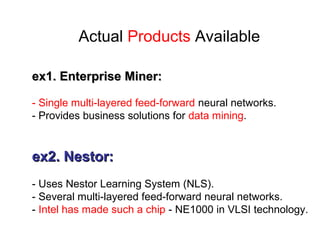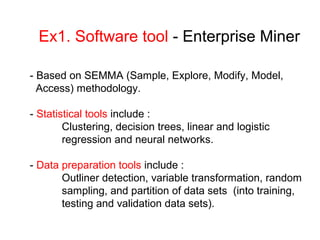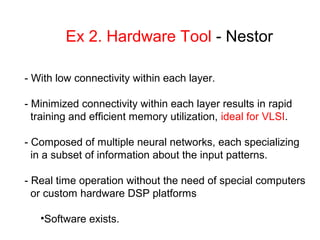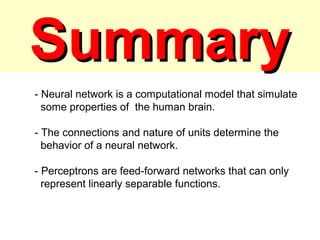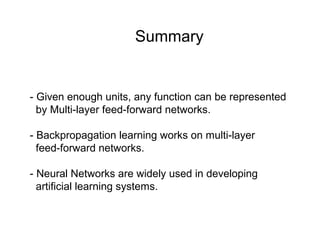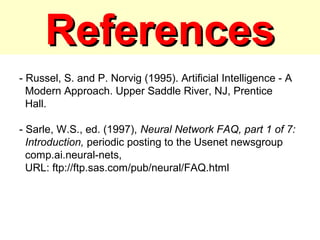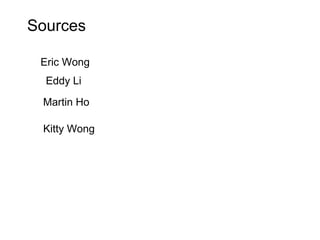1 sur 55

### Artificial neural network

• 1. ArtificialArtificial neural networkneural network Yük. Blig. Müh. Mustafa Aadel MashjalYük. Blig. Müh. Mustafa Aadel Mashjal 158229001009158229001009
• 2. Background - Neural Networks can be : - BiologicalBiological models - ArtificialArtificial models - We wish to produce artificial systems capable of complex calculation similar to the human brain.
• 3. describe the transmission ofdescribe the transmission of information and some main ideasinformation and some main ideas • The brain is consist of a mass of interconnected neurons – each neuron is connected to many other neurons • Neurons transmit signals to each other • Whether a signal is sent, depends on the strength of the bond (synapse) between two neurons
• 4. How Does the Brain Work ? (1) NEURON - It is the cell that performs information processing in the brain. - Nervous tissue its consist of neurons, which receive and transmit impulses
• 5. Each consists of : SOMA, DENDRITES, AXON, and SYNAPSE. How Does the Brain Work ? (2)
• 6. Brain vs. Digital Computers (1) - Computers require hundreds of cycles to simulate a firing of a neuron. - The brain can fire all the neurons in a single step. ParallelismParallelism - Serial computers require billions of cycles to perform some tasks but the brain takes less than a second. e.g. Face Recognition
• 7. Definition of Neural Network A Neural Network is a system that consist of many simple processing elements operating in parallel which can achieve, store, and use experimental knowledge.
• 9. Neurons vs. Units (1) - Each element of NN is a node called unit. - Units are connected by links. -Each link has a numeric weight.
• 10. Neurons vs units (2) • ANNs incorporate the two fundamental components of biological neural nets: 1. Neurones (nodes) 2. Synapses (weights)
• 11. 1- A set of connecting links, each link characterized by a weight: W1, W2, …, Wm 2- An adder function (linear combiner) which computes the weighted sum of the inputs: 3- Activation function (squashing function) for limiting the amplitude of the output of the neuron. ∑= = m 1 jjxwnetj j Structure of a nodeStructure of a node
• 12. ActivationActivation Functions - Use different functions to obtain different models. - 3 most common choices : 1) Step function 2) Sign function 3) Sigmoid function - An output of 1 represents firing of a neuron down the axon.
• 15. Feed-Forward Neural Network Architectures The feed-forward neural network was the first and most simple type of artificial neural network devised. In this network the information moves in only one direction—forward: From the input nodes data goes through the hidden nodes (if any) and to the output nodes. There are no cycles or loops in the network. • Two different classes of network architectures – single-layer feed-forward neurons are organized – multi-layer feed-forward in acyclic layers • The architecture of a neural network is linked with the learning algorithm used to train
• 16. Single Layer Feed-forward The simplest kind of neural network is a single-layer perceptron network, which consists of a single layer of output nodes; the inputs are fed directly to the outputs via a series of weights. In this way it can be considered the simplest kind of feed- forward network. The sum of the products of the weights and the inputs is calculated in each node, and if the value is above some threshold the neuron fires and takes the activated value; otherwise it takes the deactivated value. Neurons with this kind of activation function are also called artificial neurons or linear threshold units.
• 18. This class of networks consists of multiple layers of computational units, usually interconnected in a feed-forward way. Each neuron in one layer has directed connections to the neurons of the next layer. In many applications the units of these networks apply a sigmoid function as an activation function Multi Layer Feed-forward
• 19. Supervised Learning Algorithm • The learning algorithm would fall under this category if the desired output for the network is also provided with the input while training the network. By providing the neural network with both an input and output pair it is possible to calculate an error based on it's target output and actual output. It can then use that error to make corrections to the network by updating it's weights. • Single Layer neural network can be trained by a simple learning algorithm that is usually called the Delta rule. • Multi Layer neural network can be trained by a learning algorithm that is usually called the Bakpropgation.
• 20. Delta rule  The delta rule is specialized version of backpropagation's learning rule, for use with single layer neural networks.  It calculates the errors between calculated output and sample output data, and uses this to create a modification to the weights, thus implementing a form of gradient descent.  t (target) , o (output) Mu (learning rate) , beta (error) , x (input) collect old weight with the new modification weight and thus changing the networks weight
• 21. Unsupervised Learning Algorithm In this paradigm the neural network is only given a set of inputs and it's the neural network's responsibility to find some kind of pattern within the inputs provided without any external aid.
• 22. Hebb Rule Hebb method is used in the learning of networks that use Unsupervised Learning , and the weight is modify by the equation: Mu (learning rate) , o (output) , x (input) Then collect the output with the initial weight :
• 23. Introduction toIntroduction to BackpropagationBackpropagation - In 1969 a method for learning in multi-layer network, Backpropagation, was invented by Bryson and Ho. - Backpropagation is generalization of the delta rule to multi-layered feedforward networks. - Backpropagation is a common method of training artificial neural networks used in conjunction with an optimization method such as gradient descent (a first-order repeated optimization algorithm). It calculates the gradient of a loss function with respect to all the weights in the network, so that the gradient is fed to the optimization method which in turn uses it to update the weights, in an attempt to minimize the loss function.
• 24. Backpropagation LearningBackpropagation Learning DetailsDetails • Use gradient descent to minimize the error – propagate deltas to adjust for errors backward from outputs to hidden layers to inputs
• 25. Backpropagation Algorithm – Main Idea – error in hidden layers The ideas of the algorithm can be summarized as follows : 1. Computes the error term for the output units using the observed error. 2. From output layer, repeat - propagating the error term back to the previous layer and - updating the weights between the two layers until the earliest hidden layer is reached.
• 26. Forward Propagation of ActivityForward Propagation of Activity • Step 1: Initialise weights at random, choose a learning rate η • Until network is trained: • For each training example i.e. input pattern and target output(s): • Step 2: Do forward pass through net (with fixed weights) to produce output(s) – i.e., in Forward Direction, layer by layer: • Inputs applied • Multiplied by weights • Summed • ‘Squashed’ by sigmoid activation function • Output passed to each neuron in next layer – Repeat above until network output(s) produced
• 28. Step 3. Back-Step 3. Back- propagation ofpropagation of errorerror Backprop output layer
• 32. bias neuron in input layer Bias Neurons inBias Neurons in BackpropBackpropagationagation LearningLearning
• 33. Least-Mean-Square (LMS)Least-Mean-Square (LMS) AlgorithmAlgorithm Least mean squares (LMS) algorithms are a class of adaptive filter used to simulate a required filter by finding the difference between the desired and the actual signal. It was invented in 1960 by Stanford University professor Bernard Widrow .
• 38. Neural Network in Practice NNs are used for classification and function approximation or mapping problems which are: - Tolerant of some imprecision. - Have lots of training data available. - Hard and fast rules cannot easily be applied.
• 39. NETalk (1987) • Mapping character strings into phonemes so they can be pronounced by a computer • Neural network trained how to pronounce each letter in a word in a sentence, given the three letters before and three letters after it in a window • Output was the correct phoneme • Results – 95% accuracy on the training data – 78% accuracy on the test set
• 40. Other Examples • Neurogammon (Tesauro & Sejnowski, 1989) – Backgammon learning program • Speech Recognition (Waibel, 1989) • Character Recognition (LeCun et al., 1989) • Face Recognition (Mitchell)
• 41. ALVINNALVINN • Steer a van down the road – 2-layer feedforward • using backpropagation for learning – Raw input is 480 x 512 pixel image 15x per sec – Color image preprocessed into 960 input units – 4 hidden units – 30 output units, each is a steering direction
• 42. Neural Network Approaches ALVINN - Autonomous Land Vehicle In a Neural Network
• 43. Learning on-the- fly • ALVINN learned as the vehicle traveled – initially by observing a human driving – learns from its own driving by watching for future corrections – never saw bad driving • didn’t know what was dangerous, NOT correct • computes alternate views of the road (rotations, shifts, and fill-ins) to use as “bad” examples – keeps a buffer pool of 200 pretty old examples to avoid overfitting to only the most recent images
• 44. Feed-forward vs. Interactive Nets • Feed-forward – activation propagates in one direction – We usually focus on this • Interactive – activation propagates forward & backwards – propagation continues until equilibrium is reached in the network – We do not discuss these networks here, complex training. May be unstable.
• 45. Ways of learning with an ANN • Add nodes & connections • Subtract nodes & connections • Modify connection weights – current focus – can simulate first two • I/O pairs: – given the inputs, what should the output be? [“typical” learning problem]
• 46. More Neural NetworkMore Neural Network ApplicationsApplications - May provide a model for massive parallel computation. - More successful approach of “parallelizing” traditional serial algorithms. - Can compute any computable function. - Can do everything a normal digital computer can do. - Can do even more under some impractical assumptions.
• 47. Neural Network Approaches to driving - Developed in 1993. - Performs driving with Neural Networks. - An intelligent VLSI image sensor for road following. - Learns to filter out image details not relevant to driving. Hidden layer Output units Input units •Use special hardware •ASIC •FPGA •analog
• 48. Neural Network Approaches Hidden Units Output unitsInput Array
• 49. Actual Products Available ex1. Enterprise Miner:ex1. Enterprise Miner: - Single multi-layered feed-forward neural networks. - Provides business solutions for data mining. ex2. Nestor:ex2. Nestor: - Uses Nestor Learning System (NLS). - Several multi-layered feed-forward neural networks. - Intel has made such a chip - NE1000 in VLSI technology.
• 50. Ex1. Software tool - Enterprise Miner - Based on SEMMA (Sample, Explore, Modify, Model, Access) methodology. - Statistical tools include : Clustering, decision trees, linear and logistic regression and neural networks. - Data preparation tools include : Outliner detection, variable transformation, random sampling, and partition of data sets (into training, testing and validation data sets).
• 51. Ex 2. Hardware Tool - Nestor - With low connectivity within each layer. - Minimized connectivity within each layer results in rapid training and efficient memory utilization, ideal for VLSI. - Composed of multiple neural networks, each specializing in a subset of information about the input patterns. - Real time operation without the need of special computers or custom hardware DSP platforms •Software exists.
• 52. SummarySummary - Neural network is a computational model that simulate some properties of the human brain. - The connections and nature of units determine the behavior of a neural network. - Perceptrons are feed-forward networks that can only represent linearly separable functions.
• 53. Summary - Given enough units, any function can be represented by Multi-layer feed-forward networks. - Backpropagation learning works on multi-layer feed-forward networks. - Neural Networks are widely used in developing artificial learning systems.
• 54. ReferencesReferences - Russel, S. and P. Norvig (1995). Artificial Intelligence - A Modern Approach. Upper Saddle River, NJ, Prentice Hall. - Sarle, W.S., ed. (1997), Neural Network FAQ, part 1 of 7: Introduction, periodic posting to the Usenet newsgroup comp.ai.neural-nets, URL: ftp://ftp.sas.com/pub/neural/FAQ.html
• 55. Eddy Li Eric Wong Martin Ho Kitty Wong Sources

### Notes de l'éditeur

1. Teacher values were gaussian with variance 10